# Bar charts in Matplotlib

Martin McBride, 2022-03-25
Tags matplotlib bar chart
Categories matplotlib numpyIn this section, we will plot the monthly average temperatures in the UK for 2009.

This data is available in the file 2009-temp-monthly.csv. The file contains 12 entries, each representing the average of the maximum temperature of each day in the month.

We will see how to:

• Plot the data as a bar chart.
• Add month labels to the bar chart.
• Create a horizontal bar chart.

## Creating a bar chart

We have previously plotted this data using a line plot. Line plots aren't ideal for this type of data, because the line implies that there is some gradual change from one value to the next. In fact, months are discrete (or categorical) values. For example, the average temperature for January and the average temperature for February are separate values, you cannot gradually move from one to the other as the line plot implies.

A bar chart is a better way to show this information. We can create a bar chart like this:

import matplotlib.pyplot as plt
import csv

with open("2009-temp-monthly.csv") as csv_file:
temperature = [x for x in csv_reader]

months = range(12)

plt.title("Monthly temperature 2009")
plt.xlabel("Month")
plt.ylabel("Temperature")

plt.bar(months, temperature)
plt.show()


This code is available on github as barchart-monthly-temperatures.py.

All we have done here is change to using the plt.bar function instead of the plt.plot function. Here is the result: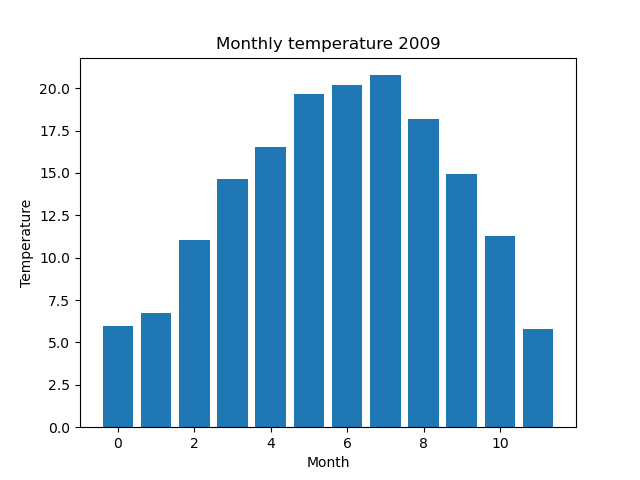## Labelling the months

The months in the plot above are numbered 0 to 11, which isn't particularly user-friendly. Here, we will see how to give them initials to make the graph a bit more readable.

To do this, we need to create a list of names for the months and use the plt.xticks function to tell Matplotlib to use those names as the labels for the ticks on the x-axis.

Here is the code:

import matplotlib.pyplot as plt
import csv

with open("2009-temp-monthly.csv") as csv_file:
temperature = [x for x in csv_reader]

month_names = ["J", "F", "M", "A", "M", "J",
"J", "A", "S", "O", "N", "D"]

months = range(12)

plt.title("Monthly temperature 2009")
plt.xlabel("Month")
plt.ylabel("Temperature")
plt.xticks(months, month_names)

plt.bar(months, temperature)
plt.show()


This code is available on github as barchart-monthly-temperatures-names.py.

Here, month_names is just a list of the labels we want to use, from 'J' for January through to 'D' for December. months is our original month numbers 0 to 11.

We pass months and month_names into plt.xticks to tell Matplotlib to use the names instead of the corresponding numbers. Here is the result: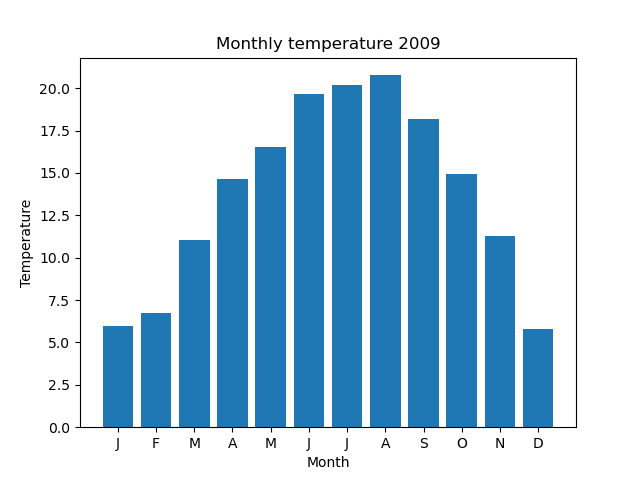## Horizontal bar charts

While we are looking at bar charts, it is also sometimes useful to make a horizontal bar chart, that is one where the bars go across the page, like this: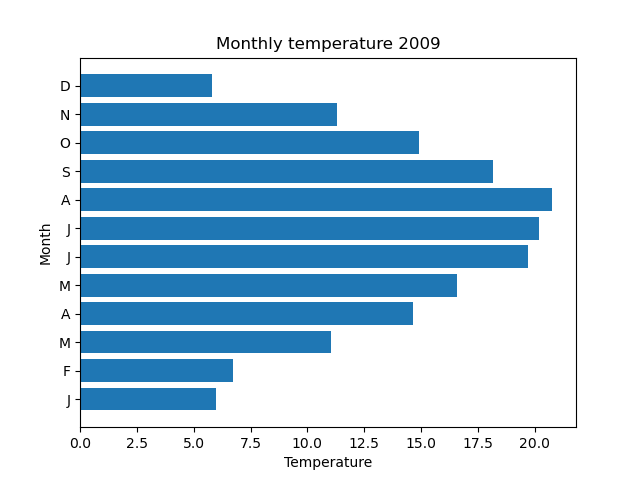This doesn't require any major changes to the code:

import matplotlib.pyplot as plt
import csv

with open("2009-temp-monthly.csv") as csv_file:
temperature = [x for x in csv_reader]

months = range(12)

month_names = ["J", "F", "M", "A", "M", "J",
"J", "A", "S", "O", "N", "D"]

plt.title("Monthly temperature 2009")
plt.xlabel("Temperature")
plt.ylabel("Month")
plt.yticks(months, month_names)

plt.barh(months, temperature)
plt.show()


This code is available on github as barchart-monthly-temperatures-h.py.

We use plt.barh rather than plt.bar. That automatically creates a horizontal bar chart.

We also need to make a couple of minor changes to the labelling. We swap the axis labels (the x-axis is now temperature and the y-axis is months). We also need to use the plt.yticks function to alter the y-axis names (rather than the x-axis names).

## Bar chart with two data sets

Here is a bar chart showing the temperatures for 2009 and 2010: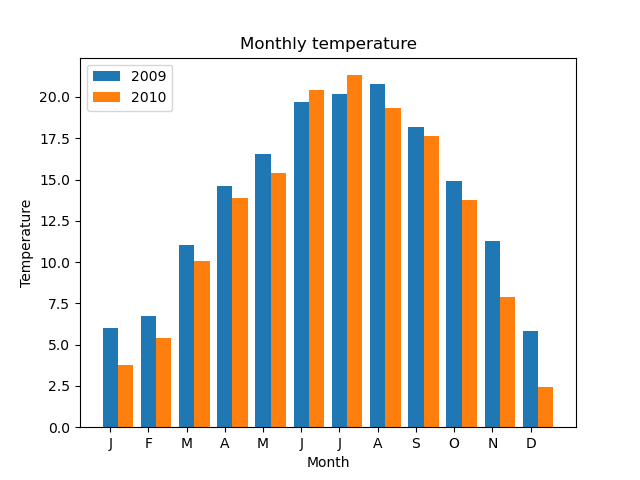And here is the code to create it:

import matplotlib.pyplot as plt
import csv

with open("2009-temp-monthly.csv") as csv_file:
temperature_2009 = [x for x in csv_reader]

with open("2010-temp-monthly.csv") as csv_file:
temperature_2010 = [x for x in csv_reader]

month_names = ["J", "F", "M", "A", "M", "J",
"J", "A", "S", "O", "N", "D"]

width = 0.4
months0 = range(12)
months1 = [x + width for x in range(12)]

plt.title("Monthly temperature")
plt.xlabel("Month")
plt.ylabel("Temperature")

plt.bar(months0, temperature_2009, width, label="2009")
plt.bar(months1, temperature_2010, width, label="2010")
plt.xticks(months0, month_names)

plt.legend(loc="upper left")

plt.show()


This code is available on github as 2year-barchart-monthly-temperatures.py.

This code is similar to the earlier barcode, with the necessary changes to create two plots:

• Reading in two sets of data.
• Calling plt.bar twice, once for each data set.
• Creating a legend to indicate the year and its corresponding colour.

For a bar chart, there is one extra important step. If we simply called plt.bar twice, then the second bar chart would be printed on top of the first bar chart, like this: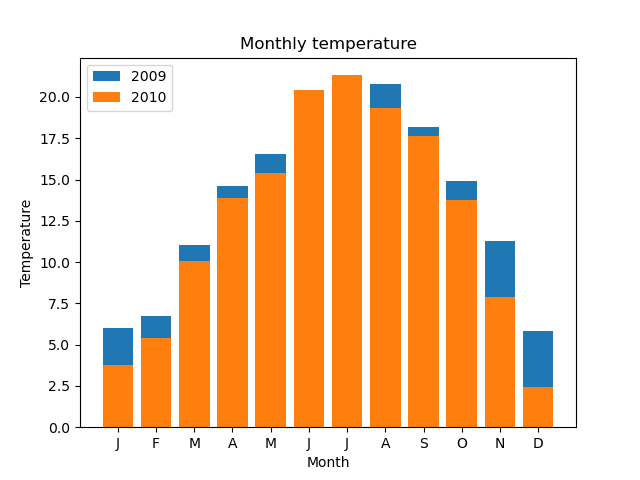That is no good. The orange bar obscures part of the blue bar. In cases where the orange bar is taller (ie when the 2010 temperature was higher than the 2009 temperature for a given month), you cannot see the blue bar at all.

We need to position the bars side-by-side, as shown in the earlier, correct bar chart. To do this, we first need to understand how Matplotlib positions the bars, which we will look at next.

## Bar positions

For a single data set, we plot a bar chart like this:

plt.bar(months0, temperature_2009)


Here, months0 is a list [0, 1, 2, 3 ... ] that serves as an x-value for each bar. So the first bar starts at x position 0, the next bar starts at x position 1, and so on. The width of each bar defaults to 0.8, so the result looks like this: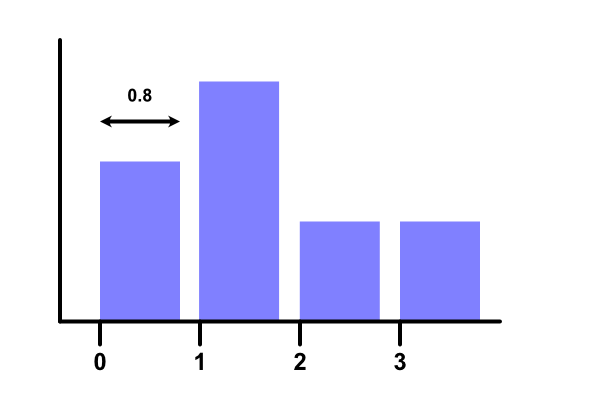To plot two bars, we must first make each bar narrower, like this:

width = 0.4
plt.bar(months0, temperature_2009, width)


plt.bar accepts a third parameter that sets the width to 0.4. Here is the resulting chart: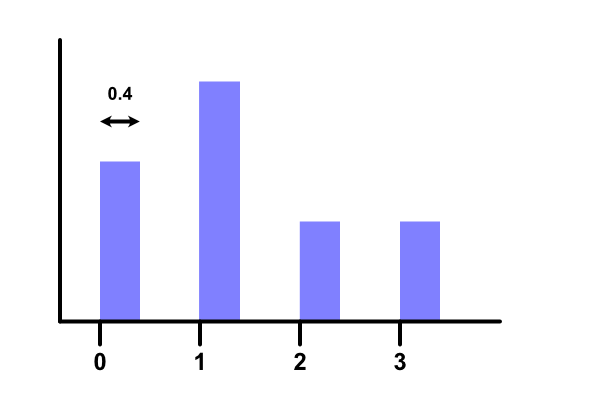Now we have created space for the second bar, but how do we position it? The first bars were positioned at 0, 1, 2..., these second bars need to be placed at 0.4, 1.4, 2.4 ... instead.

So we use a list comprehension to create a new list:

months1 = [x + width for x in range(12)]


This list contains every element in months0, but with 0.4 added to it. We plot it like this:

plt.bar(months1, temperature_2010, width)


Here is the result: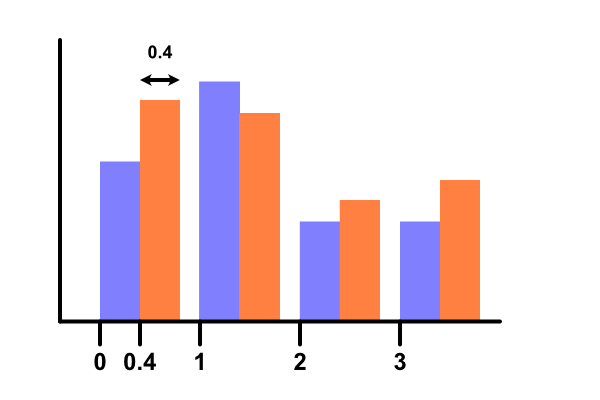If you found this article useful, you might be interested in the course Introduction to Matplotlib by the same author.

#### Popular tags

2d arrays abstract data type alignment and angle animation arc array arrays bar chart bar style behavioural pattern bezier curve built-in function callable object chain circle classes clipping close closure cmyk colour combinations comparison operator comprehension context context manager conversion count creational pattern data science data types decorator design pattern device space dictionary drawing duck typing efficiency ellipse else encryption enumerate fill filter font font style for loop function function composition function plot functools game development generativepy tutorial generator geometry gif global variable gradient greyscale higher order function hsl html image image processing imagesurface immutable object in operator index inner function input installing iter iterable iterator itertools join l system lambda function len lerp line line plot line style linear gradient linspace list list comprehension logical operator lru_cache magic method mandelbrot mandelbrot set map marker style matplotlib monad mutability named parameter numeric python numpy object open operator optimisation optional parameter or pandas partial application path pattern permutations pie chart polygon positional parameter print pure function python standard library radial gradient range recipes rectangle recursion reduce regular polygon repeat rgb rotation roundrect scaling scatter plot scipy sector segment sequence setup shape singleton slice slicing sound spirograph sprite square str stream string stroke structural pattern subpath symmetric encryption template text text metrics tinkerbell fractal transform translation transparency triangle truthy value tuple turtle unpacking user space vectorisation webserver website while loop zip